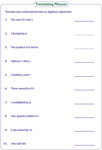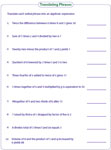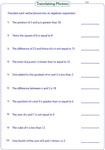1. Math Worksheets>
2. Algebra>
3. Translating Phrases

# Translating Phrases into Algebraic Expressions Worksheets

The worksheets in this page provide practice to students on translating phrases into algebraic expressions like linear expressions, single & multiple variable expressions, equations and inequalities. This will help the students to translate real-life problems into algebraic expressions and find a solution in an easier way.

Linear Algebraic Expressions

Translate each phrase into a linear algebraic expression. Each expression is in the form of ax + b, where x is any variable; a and b are constants. Difficult level is in the form of c(ax+b), c is a constant.Easy:

Moderate:

Difficult:

Single Variable Algebraic Expressions

The worksheets contain phrases, which need to be translated into algebraic expressions. Each expression is in the form of f(x) + c, where f(x) is a polynomial in different forms, c is a constant.Easy:

Moderate:

Difficult:

Multi-variables Algebraic Expressions

Each worksheet has 10 verbal phrases, which should be translated into algebraic expressions. An expression involves two or more variables with or without a constant.Easy:

Moderate:

Translating Linear Equations: One-Step

Translate each phrase into one-step linear equation. The worksheets are classified into easy and moderate levels.Easy:

Moderate:

Translating Linear Equations: Two-Step

Identify the keywords and translate the sentences into two-step linear equations.Easy:

Moderate:

Translating Linear Equations: Multi-Step

Set up the multi-step equations by translating the verbal phrases. This will help students to solve multi-step equations involving word problems.Easy:

Moderate:

Translating Linear Inequalities

Translate the phrases into inequalities, which involve a linear function. The expressions include symbols like <, >, 'not equal', 'less than or equal' and 'greater than or equal'.Basic:

One-step:

Two-step (Easy):

Two-step (Moderate):

MW4K's Mixed Bag

This section contains phrases, which are a mixture of linear equations and inequalities. The worksheets are distributed in two levels: Easy and Moderate.Easy:

Moderate:

Stock up your library with the complete set of worksheets on translating phrases into algebraic expressions.

Related Worksheets